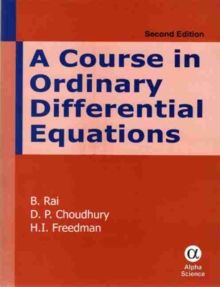Supporting your high street Find out how »
• My AccountA Course in Ordinary Differential Equations Hardback

Description

This text book for the undergraduate and postgraduate courses presupposes no background other than elementary calculus.

The novel features of the book are: systematic method of finding integrating factors of the first order equations; unified treatment of several categories of equations under the banner of Linerarly Separable equations; rational approach to Singular Solutions; sound treatment of inverse operator method for obtaining particular solutions of non-homogeneous linear equations; technique of solving systems of linear equations with constant coefficients equivalent to the use of Jordan Canonical form of the associated matrix.

Theoretically sound approach to the method of Laplace transforms; elaboration of properties of Legendre and Bessel functions and inclusion of all the major numerical techniques for solving differential equations along with a derivation of the Runge Kutta formula; and additional exercises with answers.

All the methods given in the book are explained by the help of solved examples.

It is an adequate number of unsolved exercises have also been provided on each topic.

Information

• Format: Hardback
• Pages: 490 pages
• Publisher: Alpha Science International Ltd
• Publication Date:
• Category: Differential calculus & equations
• ISBN: 9781842657720

£39.95

£33.95

on all orders

Pick up orders

from local bookshops